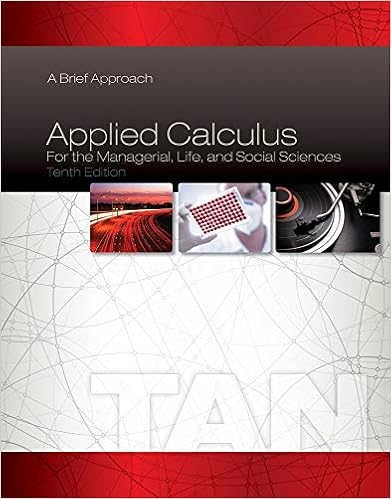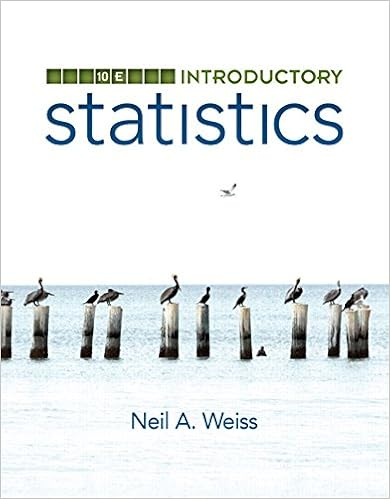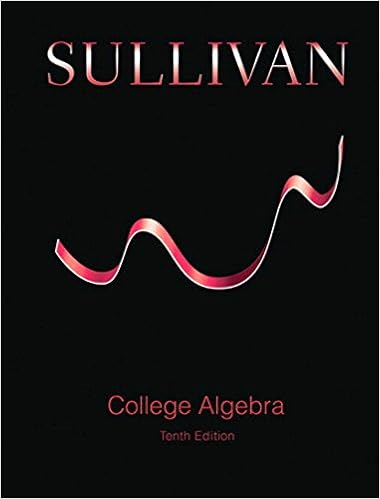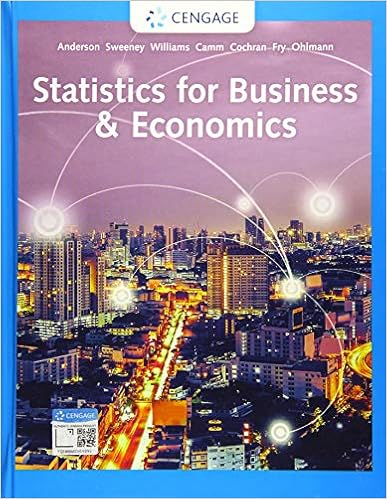# geo_analytic-geometry-classwork-homework_2015-04-08 -...

• Homework Help
• 33

This preview shows page 1 - 11 out of 33 pages.

Analytic GeometryDistance Formula:CLASSWORK1.Find the distance between (-9, 1) & (-5, -2)2.Find the distance between (10, 3) & (1, -3)3.Length of´BD= ?4.Length of´AD= ?5.Length of´DC= ?Distance Formula:HOMEWORK6.Find the distance between (2, 9) & (-3, 14)7.Find the distance between (-3, 2) & (9, 7)8.length of´AD= ?9.length of´BD= ?10.length of´CD= ?Geometry – Analytic Geometry~1~NJCTL.org#3 - 5#8 - 10
##### We have textbook solutions for you!The document you are viewing contains questions related to this textbook.
Chapter 1 / Exercise 24
Applied Calculus for the Managerial, Life, and Social Sciences: A Brief Approach
TanExpert VerifiedBrowse all Textbook Solutions
Midpoint Formula:CLASSWORKCalculate the coordinates of the midpoint of the given segmentsGeometry – Analytic Geometry~2~NJCTL.org
11.(0, 0), (6, 10)12.(2, 3), (6, 7)13.(4, -1), (-2, 5)14.(-3, 8), (13, -6)15.(-1, -14), (-2, -6)16.(3, 2), (6, 6)3
17.(-5, 2), (0, 4)Calculate the coordinates of the other endpoint of the segment with the given endpoint and midpoint M4
18.endpoint: (4,6), midpoint:(7,11)19.endpoint: (2, 6), midpoint:(-1, 1)20.endpoint: (3, -12), midpoint(2,-1)5
Midpoint Formula:HOMEWORKCalculate the coordinates of the midpoint of the given segments6
21.(0, 0), (8, 4)22.(-1, 3), (7, -1)23.(3, 5), (7, -9)24.(6, 0), (2, 7)25.(-5, -3), (-3, -5)26.(13, 8), (-6, -6)7
27.(-4, -2), (1, 3)Calculate the coordinates of the other endpoint of the segment with the given endpoint and midpoint M8
28.endpoint: (-5, 9)midpoint (-8, -2)29.endpoint: (6, 7)midpoint (10, -7)30.endpoint: (2, 4)midpoint (-1, 7)Partitions of a Line Segment:CLASSWORKPARCC-type Questions:31.Line segment AB in the coordinate plane has endpoints with coordinates A (3, -10) and B(-6, -1).a)Graph´b)Find 2 possible locations for point C so that C divides´ABinto 2 parts with lengths in a ratio of 1:2.32.Line segment EF in the coordinate plane has endpoints with coordinates E (-10, 11) and F (5, -9).a)Graph´ABEF9
b)Find 2 possible locations for point G so that G divides´EFinto 2 parts with lengths in a ratio of 2:3.33.Line segment JK in the coordinate plane has endpoints with coordinates J (11, 11) and K (-10, -10).Findtwo possible locations for point P that divides´JKinto two parts with lengths in a ratio of 3:4.34.Line segment LM in the coordinate plane has endpoints with coordinates L (-12, 10) and M (6, -8).Findtwo possible locations for point P that divides´LMinto two parts with lengths in a ratio of 2:7.35.Line segment RS in the coordinate plane has endpoints with coordinates R(7, -11) and S(-9, 13).Find twopossible locations for point P that divides´RSinto two parts with lengths in a ratio of 3:5.Partitions of a Line Segment:HOMEWORKPARCC-type Questions:36.Line segment AB in the coordinate plane has endpoints with coordinates A (5, -7) and B(-10, 3).a)Graph´b)Find 2 possible locations for point C so that C divides´ABinto 2 parts with lengths in a ratio of 1:4.AB10

Course Hero member to access this document

Course Hero member to access this document

End of preview. Want to read all 33 pages?

Course Hero member to access this document

Term
Spring
Professor
N/A
Tags
Math, Elementary geometry
##### We have textbook solutions for you!
The document you are viewing contains questions related to this textbook.The document you are viewing contains questions related to this textbook.
Chapter 1 / Exercise 24
Applied Calculus for the Managerial, Life, and Social Sciences: A Brief Approach
TanExpert Verified
•••